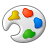# Convert Float to Double - different values

353
0
07-31-2013 11:54 PMNew Contributor
Hi,

I would like convert float field to double field. So I used Add Field with Type Double and Precision 15. Then I used Raster Calculator to assign float field to double field. The problem is in values. Float field has values e.g. 365 460 500 (always double zeros at the end - probably because of Precision), but double field has different values (e.g. 365 460 512). I know that float field is rounded (calculate from Zonal Statistics as Table), but why are these values different?